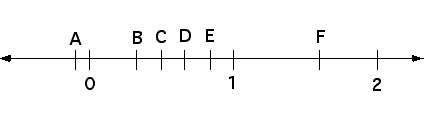# Where is that fraction?• If the fractions represented by the points D and E are multiplied, what point on the number line best represents the product?
• If the fractions represented by the points C and D are multiplied, what point on the number line best represents the product?
• If the fractions represented by the points B and F are multiplied, what point on the number line best represents the product?
• Suppose 20 is multiplied by the number represented by E on the number line. Estimate the product.
• Suppose 20 is divided by the number represented by E on the number line. Estimate the quotient.